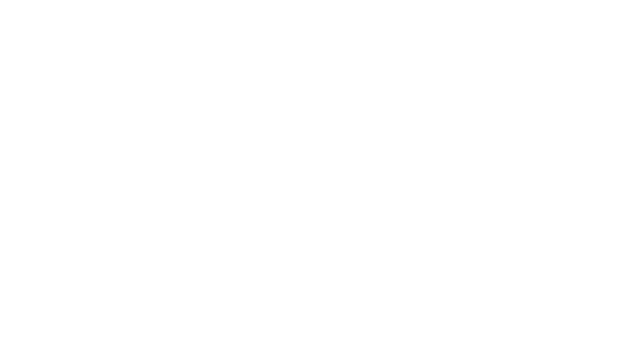## 1. 从图片分离组件区域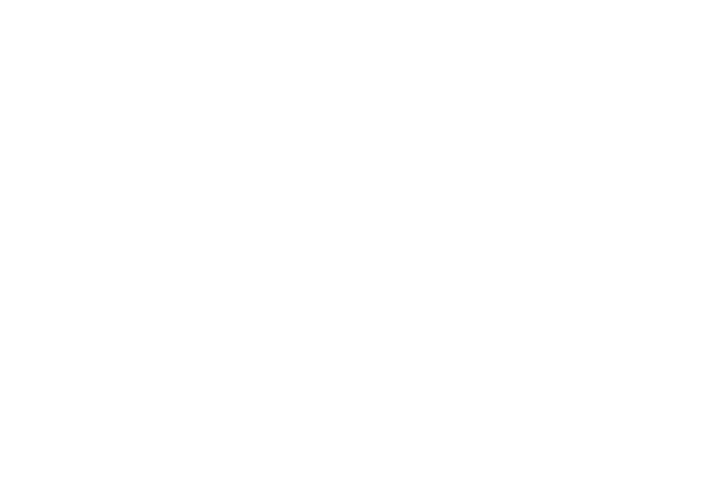### 代码实现

```def extract(img, rect):
"""输入框选区，输出GrabCut遮罩"""
x, y, w, h = rect
roi_img = img[y:y+h, x:x+h]
mask = np.zeros(roi_img.shape[:2], np.uint8) # 初始化遮罩层
bgdModel = np.zeros((1, 65), np.float64)
fgdModel = np.zeros((1, 65), np.float64)
cv2.grabCut(img, mask, rect, bgdModel, fgdModel, 4, cv2.GC_INIT_WITH_RECT)

### 2.1 外轮廓提取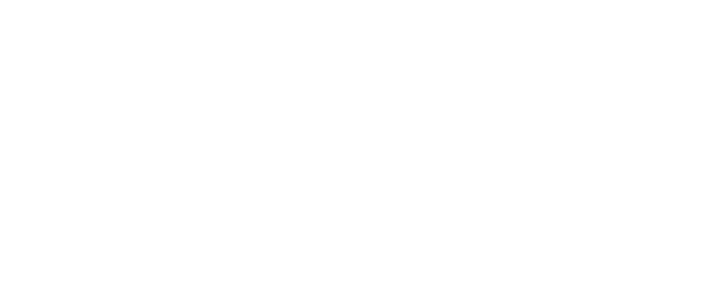### 代码实现

```def separate(img, th=5):
"""输入组件区域遮罩，输出多个组件外轮廓列表"""
new_img = img.copy()
showImg(new_img)
new_img = cv2.Canny(new_img, 50, 150)
new_img = image_morphology(new_img)
cnts, _ = cv2.findContours(new_img, cv2.RETR_EXTERNAL, cv2.CHAIN_APPROX_NONE)
data = []
for cnt in cnts:
x, y, w, h = cv2.boundingRect(cnt)
if (w < th) | (h < th):
"""剔除噪点"""
continue
data.append((cnt, x, y, w, h))
return data```

### 2.2 形状检测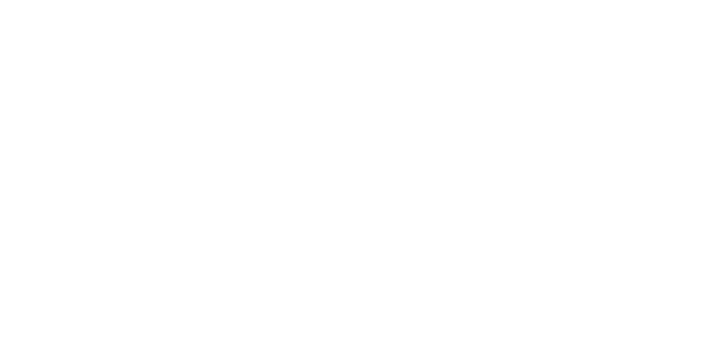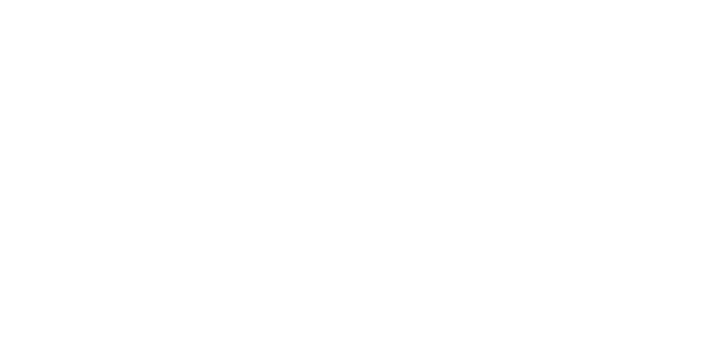### 2.2.1 矩形检测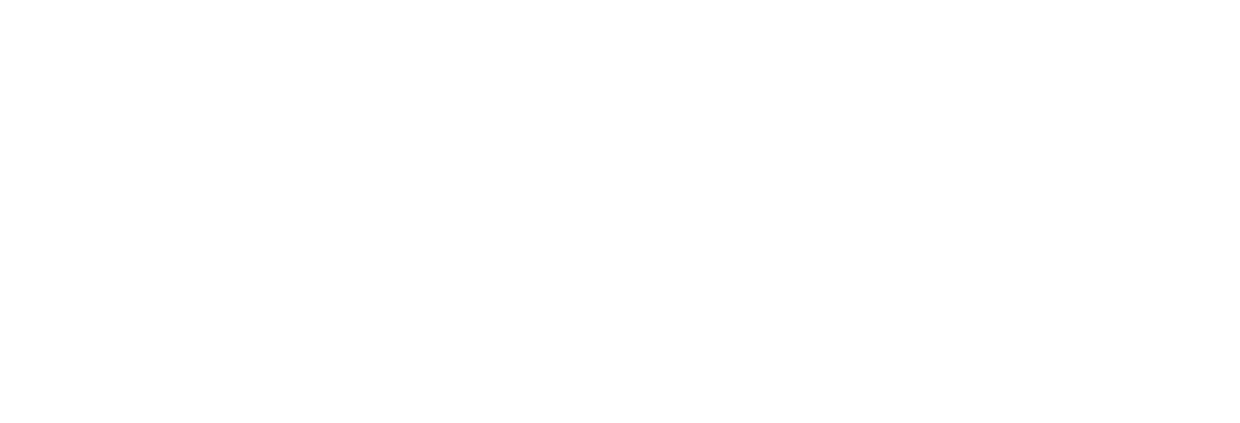OpenCV提供线段检测方法 `cv2.HoughLinesP` ，输入外轮廓，输出检测到的线段，具体代码实现如下：

```# 检测矩形
def detectRectangle(img, width, height):
minLineLength = 10
maxLineGap = 4
# 霍夫直线变换输出检测到的线段数组
lines = cv2.HoughLinesP(img, 1, np.pi/180, 100, minLineLength, maxLineGap)
segments = lines.reshape(lines.shape, 4)
# 将线段数组进行进一步检测，判断是否命中矩形规则
return judgeRectangle(segments, width, height)```

### 代码实现

```"""判断是否为矩形"""
def judgeRectangle(lines, width, height, x=0, y=0):
th = 2
horizontal_segments = lines[np.where(abs(lines[:, 1] - lines[:, 3]) < th)]
vertical_segments = lines[np.where(abs(lines[:, 0] - lines[:, 2]) < th)]
isRect = False
h = w = None
if horizontal_segments.size != 0:
horizontal_centers = (
horizontal_segments[:, 1] / 2 + horizontal_segments[:, 3] / 2
)
top = horizontal_centers.min()
bottom = horizontal_centers.max()
h = bottom - top
if abs(h - height) > th:
return False, None, None  # 如果两线间隔非图形高度，则不规则图片
isRect = True
h = int(round(h))
if vertical_segments.size != 0:
vertical_centers = vertical_segments[:, 0] / 2 + vertical_segments[:, 2] / 2
left = vertical_centers.min()
right = vertical_centers.max()
w = right - left
if abs(w - width) > th:
return False, None, None
isRect = True
w = int(round(w))
return isRect, w, h```

### 2.2.2 圆形检测

```# 检测圆形
def detectCircle(img, width, height):
if circles is None: return False
return judgeCircle(radius, rx, ry, width, height)
def judgeCircle(r, rx, ry, w, h, x=0, y=0, th=4):
return (
(abs(w - h) < th)
& (abs(r - w / 2) < th)
& (abs(rx - x - w / 2) < th)
& (abs(ry - y - h / 2) < th)
)```

## 3. 组件的样式计算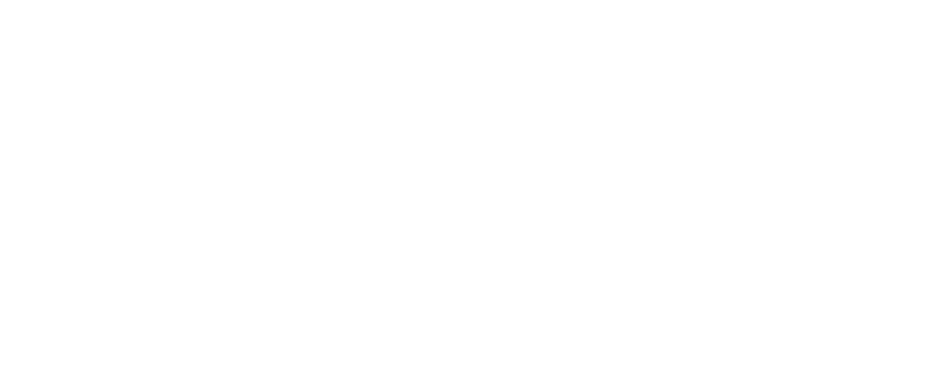### 3.1 圆角计算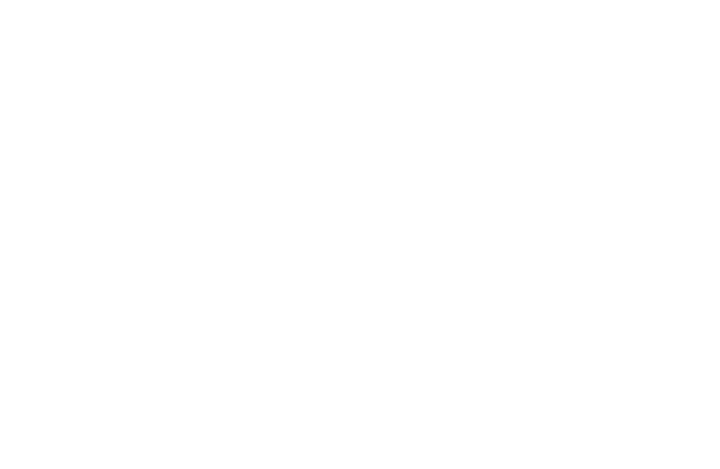1. 假设存在圆角，用面积推算圆角半径，确定“候选区域”

1. 构造“候选区域”水平-竖直轴对称图形，对图形进行霍夫圆环检测，验证是否为圆角

### 3.1.1 圆角半径推算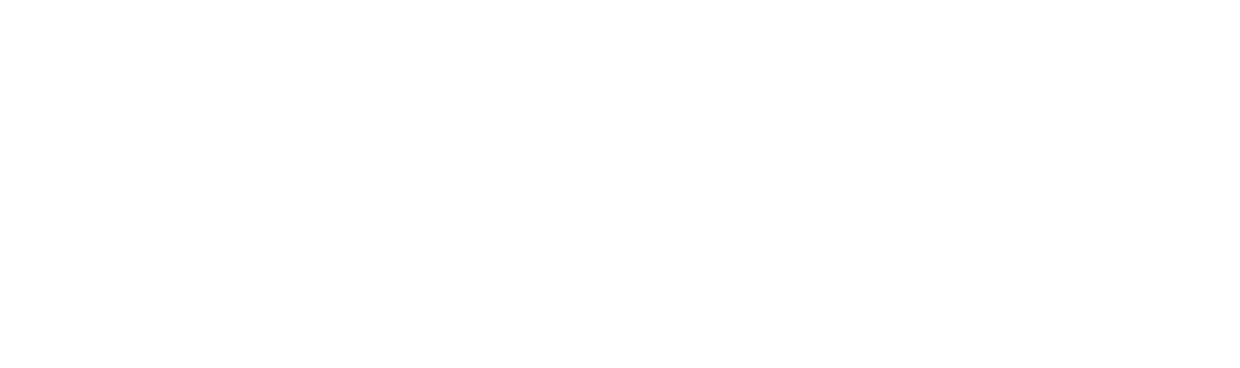### 3.1.2 候选区域验证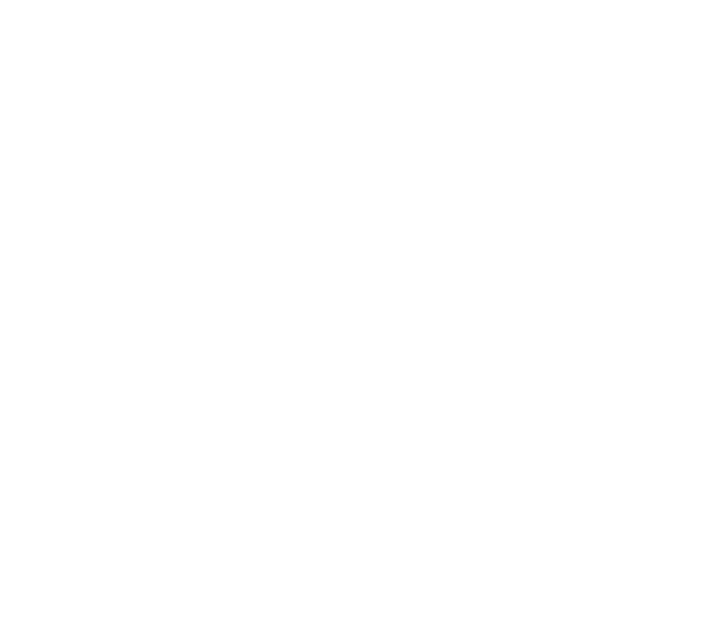### 代码实现

```# 推算可能的圆角半径
corner_mask_size = img[img[:, :, 3] != 1].size
#
# 验证候选区域是否为圆角，以左上圆角为例
binary_image = np.zeros(cornerArea.shape[0:2],dtype=np.uint8) # 构造二值图
binary_image[cornerArea[:,:,3] != 0] = 255
horizontal = cv2.flip(img, 1, dst=None) # 水平镜像
img=cv2.hconcat([img, horizontal]) # 水平拼接
showImg(img)
vertical = cv2.flip(img, 0, dst=None) # 垂直镜像
img=cv2.vconcat([img, vertical]) # 垂直拼接
img = cv2.copyMakeBorder(img, 5, 5, 5, 5, cv2.BORDER_CONSTANT, value = )
if circles is None: return False
else: return True```

### 3.2 边框计算

1. 色块分离：对图像基于颜色聚类，相近色区聚类同一色块

1. 内外轮廓相似度计算：遍历不同色块，提取每个色块内外轮廓，并计算其相似度

### 3.2.1 色块分离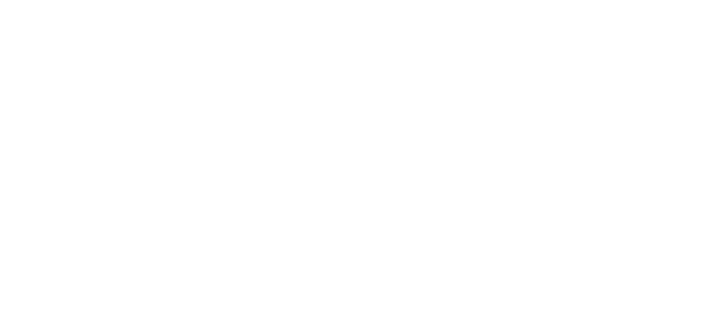### 代码实现

```"""k-means聚类"""
def image_kmeansSegement(img, k=6):
# 将图片从RGB空间转为HSV
img = cv2.cvtColor(img, cv2.COLOR_BGR2HSV)
data = img.reshape((-1, 3))
data = np.float32(data)
# MAX_ITER最大迭代次数，EPS最高精度
criteria = (cv2.TERM_CRITERIA_EPS + cv2.TERM_CRITERIA_MAX_ITER, 10, 1.0)
num_clusters = k
ret, label, center = cv2.kmeans(
data, num_clusters, None, criteria, num_clusters, cv2.KMEANS_RANDOM_CENTERS
)
center = cv2.cvtColor(np.array([center], dtype=np.uint8), cv2.COLOR_HSV2BGR)
labels = label.flatten()
return labels, center```

### 3.2.2 内外轮廓相似度计算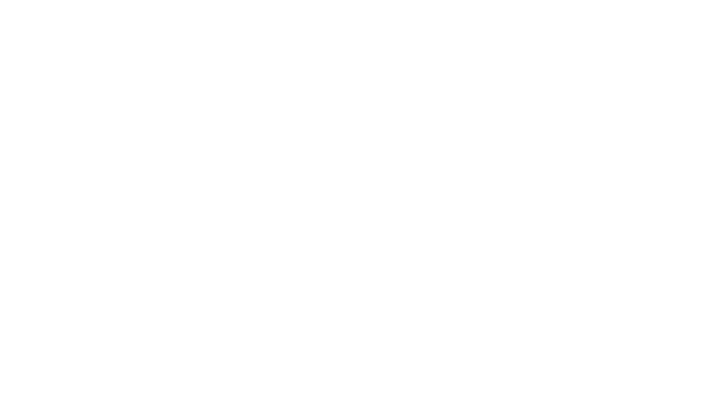### 代码实现

```"""验证每个色块是否存在边框特征B"""
def borderExtract(labels, center, img_filled):
# 遍历k-means分离的k个色块
for i in range(labels.max()):
area = np.zeros((labels.size), dtype=np.uint8)
area[labels == i] = 255
area = area.reshape(img_filled.shape)
# 获取当前色块外轮廓，用白色填充
outter_filled, *_ = image_contours(area)
# 获取当前色块内轮廓，用白色填充
result = outter_filled - area
result[result < 0] = 0
inner_filled, *_ = image_contours(result)
# 判断外轮廓和内轮廓是否相似
if isSimilar(outter_filled, inner_filled) & isSimilar(img_filled, filled1):
s1 = np.where(filled1 > 0).size
s2 = np.where(filled2 > 0).size
scale = (1.0 - math.sqrt(s2 / s1)) * 0.5
_drawBorder(filled1 - filled2, center[i])
return scale, center[i], filled2
return None
"""使用pHash算法计算轮廓之间相似度"""
def isSimilar(img1, img2, th=0.8):
HASH1 = PHash.pHash(img1)
HASH2 = PHash.pHash(img2)
distance, score = PHash.hammingDist(HASH1, HASH2)
print(score)
return score > th```